NEET  >  Test: Magnetic & Electric Properties of Solids & Semiconductors

# Test: Magnetic & Electric Properties of Solids & Semiconductors - NEET

Test Description

## 24 Questions MCQ Test Chemistry Class 12 - Test: Magnetic & Electric Properties of Solids & Semiconductors

Test: Magnetic & Electric Properties of Solids & Semiconductors for NEET 2023 is part of Chemistry Class 12 preparation. The Test: Magnetic & Electric Properties of Solids & Semiconductors questions and answers have been prepared according to the NEET exam syllabus.The Test: Magnetic & Electric Properties of Solids & Semiconductors MCQs are made for NEET 2023 Exam. Find important definitions, questions, notes, meanings, examples, exercises, MCQs and online tests for Test: Magnetic & Electric Properties of Solids & Semiconductors below.
Solutions of Test: Magnetic & Electric Properties of Solids & Semiconductors questions in English are available as part of our Chemistry Class 12 for NEET & Test: Magnetic & Electric Properties of Solids & Semiconductors solutions in Hindi for Chemistry Class 12 course. Download more important topics, notes, lectures and mock test series for NEET Exam by signing up for free. Attempt Test: Magnetic & Electric Properties of Solids & Semiconductors | 24 questions in 35 minutes | Mock test for NEET preparation | Free important questions MCQ to study Chemistry Class 12 for NEET Exam | Download free PDF with solutions
 1 Crore+ students have signed up on EduRev. Have you?
Test: Magnetic & Electric Properties of Solids & Semiconductors - Question 1

### Only One Option Correct Type This section contains 7 multiple choice questions. Each question has four choices (a), (b), (c) and (d), out of which ONLY ONE is correct. Consider the following species. I. Mg(s) II. TiO(s) III. I2(s) IV. H2O (s) Q. Which of the above solids is/are electrical conductor?

Detailed Solution for Test: Magnetic & Electric Properties of Solids & Semiconductors - Question 1

I. Mg (metal) conducts electricity in solid state as well as in molten state due to available free valence electrons.

II. TiO behaves like metals and is a good conductor of electricity.

III. I2 is a bad conductor.

IV. H2O (ice) is a bad conductor.

Test: Magnetic & Electric Properties of Solids & Semiconductors - Question 2

### Which kind of defects are introduced by doping?

Detailed Solution for Test: Magnetic & Electric Properties of Solids & Semiconductors - Question 2

(a) When smaller cation migrates to interstitial site, it is called dislocation defect. It is also called Frenkel defect.

(b) When equal number of cation and anion are missing or one cation (A+) is missing and charge is balanced by A2+ , then this is called Schottky defect.

(c) as in (a)

(d) Electronic defects (n-type, p -type) is due to doping of impurity.

Test: Magnetic & Electric Properties of Solids & Semiconductors - Question 3

### Silicon doped with electron-rich impurity forms

Detailed Solution for Test: Magnetic & Electric Properties of Solids & Semiconductors - Question 3

(b)

(a) When Si is doped with electron-deficient impurity -(p -type)

(b) When Si is doped with electron-rich impurity-(n-type)

(c) In case of semiconductors, the gap between the valence band and conduction band is small. Some electrons may Jump to conduction band and thus, conduction is observed. Semiconductors of this type are called intrinsic semiconductors.

(d) Insulators are non-conducting with high energy gap.

Test: Magnetic & Electric Properties of Solids & Semiconductors - Question 4

Select the correct matching.

Detailed Solution for Test: Magnetic & Electric Properties of Solids & Semiconductors - Question 4

(c)

When polar crystal is subjected to a mechanical stress, electricity is produced. It is called piezoelectricity.

Piezoelectric crystals with permanent dipoles (domains aligned in ! same direction) are said to have ferroelectricity as in (a) and with : zero dipoles (domains aligned equally in opposite direction) are said to have anti-ferroelectricity as in (b).

Test: Magnetic & Electric Properties of Solids & Semiconductors - Question 5

Which of the following oxides behaves as conductor or insulator depending upon temperature?

Detailed Solution for Test: Magnetic & Electric Properties of Solids & Semiconductors - Question 5

Metals are good conductors of electricity and have conductivities in the order 107(Ωm-1) or 105(Ωm-1).
Insulator: Solids having range of conductivity from 10-20 to 10-10 ohm-1 m-1 are considered as insulators.
Semiconductor: Solids having intermediate range of conductivity, i.e. from 10-6 to 104 ohm-1 m-1 are called semiconductors.

Test: Magnetic & Electric Properties of Solids & Semiconductors - Question 6

How many energy levels are present in 3s conduction band of a single crystal of sodium weighing 26.8 mg?

Detailed Solution for Test: Magnetic & Electric Properties of Solids & Semiconductors - Question 6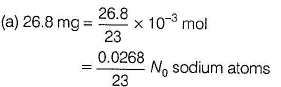Electrons in 3 s orbital in one sodium atom (conduction band) = 1

Thus, total conduction bands (energy levels =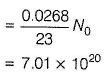Test: Magnetic & Electric Properties of Solids & Semiconductors - Question 7

In which of the following oxides conducting or insulating property of is dependent on temperature?

Detailed Solution for Test: Magnetic & Electric Properties of Solids & Semiconductors - Question 7

Ti2O3, conducting below 41OK and insulating at about 150 K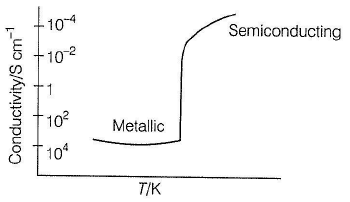*Multiple options can be correct
Test: Magnetic & Electric Properties of Solids & Semiconductors - Question 8

One or More than One Options Correct Type
This section contains 5 multiple type questions. Each question has 4 choices (a), (b), (c) and (d), out of which ONE or MORE THAN ONE are correct.

Select the correct statement(s) about the movement of electrons and holes in a p-type semiconductor when an electric field is applied.

Detailed Solution for Test: Magnetic & Electric Properties of Solids & Semiconductors - Question 8

(a,b) When Si or Ge is doped with a group 13 elements (B, Al, Ga) then, one valence electron of Si makes a hole or vacancy. When electric field is applied, electrons move towards positively charged plate through electronic holes. It would appear that electronic holes are positively charged and are moving towards negatively charged plate.

*Multiple options can be correct
Test: Magnetic & Electric Properties of Solids & Semiconductors - Question 9

Select the correct statement(s).

Detailed Solution for Test: Magnetic & Electric Properties of Solids & Semiconductors - Question 9

(a,b,c,d)

Si ( 1 4 ) - [Ne] 3s2 3p2 (group 14 element having four valence electrons). Thus, each atom of Si is covalently bonded to four neighbouring atoms.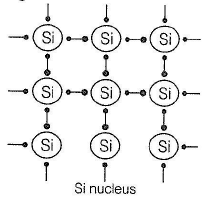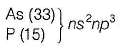3 (five valence electrons

Group 15 elements Four electrons are involved in bonding with | four electrons of Si and one remaining electron becomes I delocalised and increases the conductivity of Si. Thus, increase in I the conductivity is due to doped negatively charged electron. This is called n-type semiconductor.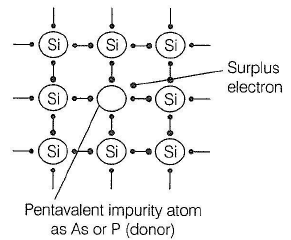Group 13 elements

[B, Al, Ga] ns2np1 In this case, B, Al or Ga is short of one electron (as compared to Si). This is called electron hole or electron vacancy. Under the influence of electric field, electron hole moves towards the positively charged plate and is called p -type semiconductor.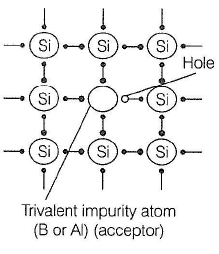Test: Magnetic & Electric Properties of Solids & Semiconductors - Question 10

Which of the following oxides behaves as conductor or insulator depending upon temperature?

Detailed Solution for Test: Magnetic & Electric Properties of Solids & Semiconductors - Question 10

The correct answer is option C
TiO3​ behaves as conductor or insulator depending on temperature because of a variation of energy gap between valence band and conduction band with the variation of temperature.

*Multiple options can be correct
Test: Magnetic & Electric Properties of Solids & Semiconductors - Question 11

Select the correct statement(s).

Detailed Solution for Test: Magnetic & Electric Properties of Solids & Semiconductors - Question 11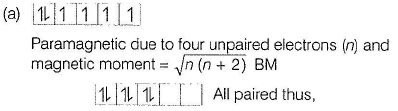n = 0, Magnetic moment = 0

Thus, (a) is correct.

(b) Paramagnetic substances are magnetised in a magnetic field in the same direction hence, weakly attracted thus, (b) is correct.

(c) Ferromagnetic substances can be magnetised permanently, thus, given statement is incorrect.

(d) Substances like MnO showing anti-ferromagnetism have domain structure similar to ferromagnetic substances, but their domains are oppositely oriented and cancel out each other's magnetic moment.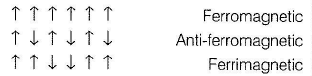*Multiple options can be correct
Test: Magnetic & Electric Properties of Solids & Semiconductors - Question 12

Germanium has been doped with impurity as shown in I, II, III, IV. Select the correct statement(s).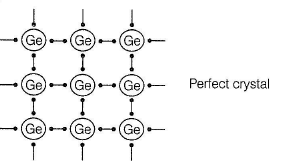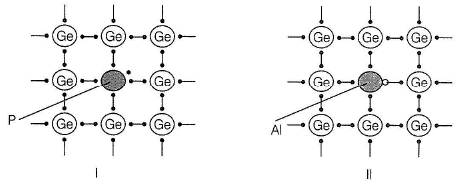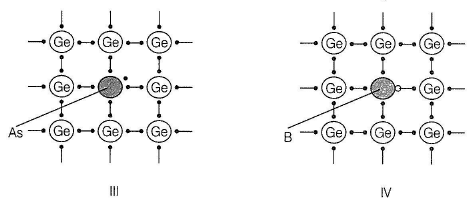Detailed Solution for Test: Magnetic & Electric Properties of Solids & Semiconductors - Question 12

(a,b)

I and III have been doped with pentavalent impurity (P and As). There is one surplus electron and this results in the formation of n-type semiconductor. Surplus electron is responsible for conduction. II and IV have been doped with trivalent impurity (B and Al).
There is one electron short for bonding with Ge, hence a hole is formed. Hole moves through the electric field. This is p-type semiconductor.

Test: Magnetic & Electric Properties of Solids & Semiconductors - Question 13

Direction (Q. Nos. 13-17) This section is based on Statement I and Statement II. Select the correct answer from the codes given below.

Q.

Statement I : Graphite is a good conductor of electricity.
Statement II : In graphite, carbon atom is sp2 hybridised.

Detailed Solution for Test: Magnetic & Electric Properties of Solids & Semiconductors - Question 13

. (b)

Graphite has four valence electron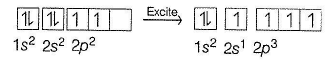Three electrons are involved in covalent bonding with neighbouring atoms giving it a hexagonal type packing (layers) such that one layer can slide over the other. The fourth electron makes the graphite conducting. Hexagonal sheets are with sp2- hybridised carbon.
Thus, both Statements I and II are correct but Statement II is not the correct explanation of Statement I.

Test: Magnetic & Electric Properties of Solids & Semiconductors - Question 14

Statement I : A ferromagnetic substance becomes a permanent magnet, when it is placed in a magnetic field.

Statement II : All the domains get oriented in the direction of magnetic field.

Detailed Solution for Test: Magnetic & Electric Properties of Solids & Semiconductors - Question 14

(a)

In solid state, metal ions of ferromagnetic substances are grouped together into small regions called domains. Each domain acts as a tiny magnet. In an unmagnetised ferromagnetic substance, domains are randomly oriented and their magnetic moments are cancelled. In a magnetic field, all the domains are oriented in the direction of the magnetic field producing a strong magnetic effect and magnetism persists permanently even after magnetic field is removed.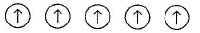Thus, both Statements I and II are correct and Statement II is the correct explanation of Statement I

Test: Magnetic & Electric Properties of Solids & Semiconductors - Question 15

Statement I : In the case of anti-ferrom agnetic substances, magnetic moment is zero.

Statement II : Domains get oriented in the direction of applied magnetic field.

Detailed Solution for Test: Magnetic & Electric Properties of Solids & Semiconductors - Question 15

(c)

Anti-ferrom agnetic substances (as MnO) have following domain arrangements

following domain arrangements.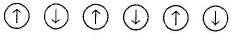These domains are oppositely oriented and cancel out each other's magnetic moment and making it zero (even in the absence of magnetic field).
Thus, Statement I is correct and Statement II is incorrect.

Test: Magnetic & Electric Properties of Solids & Semiconductors - Question 16

Statement I : The electrical conductivity of a metal decreases as temperature increases.

Statement II : This impedes the flow of electrons, when an electric field is applied.

Detailed Solution for Test: Magnetic & Electric Properties of Solids & Semiconductors - Question 16

(b)

Increase in temperature causes thermal agitation of metal ions and thus, electrical conductivity is decreased. Thus, Statement I is correct. In the presence of electric field, flow of electrons is impeded. Thus, Statement II is correct but is not the correct explanation of Statement I.

Test: Magnetic & Electric Properties of Solids & Semiconductors - Question 17

Statement I : Electrical conductivity of semiconductors increases with rise in temperature.

Statement II : There is a small energy gap between conduction band and valence band.

Detailed Solution for Test: Magnetic & Electric Properties of Solids & Semiconductors - Question 17

(b) Sem iconductors have small energy g ap between conduction band and valence band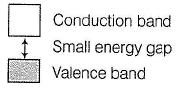On increasing temperature, electron from valence band can jump to conduction band and thus, electrical conductivity is increased. Thus, both Statements I and II are correct but Statement II is not the correct explanation of Statement I.

Test: Magnetic & Electric Properties of Solids & Semiconductors - Question 18

Direction (Q . No. 18) Choices for the correct combination of elements from Column I and Column II are given as options (a), (b), (c) and (d), out of which one is correct.

Q.

Match the items in Column I with the terms in Column II and select the answer from the codes given: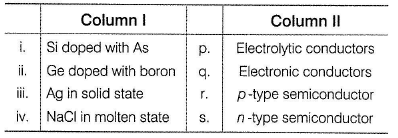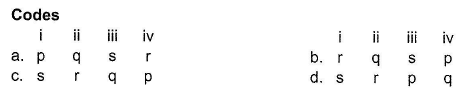Detailed Solution for Test: Magnetic & Electric Properties of Solids & Semiconductors - Question 18

(i) Si doped with As has one extra electron for conduction, thus n-type semiconductor.

(ii) Ge doped with B has short of one electron thus, a hole is formed, thus a p-type semiconductor.

(iii) Metals have sea of electrons responsible for electronic conduction.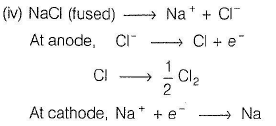Electron flow is due to electrolysis. Thus, electrolytic conduction.
Thus, (i) (s),

(ii) -> (r),

(iii) -» (q),

(iv) (p).

Test: Magnetic & Electric Properties of Solids & Semiconductors - Question 19

Direction (Q. Nos. 19 and 20) This section contains 1 paragraph, which describing theory, experiments, data, etc. Two questions related to the paragraph have been given. Each question has only one correct answer among the four given options (a), (b), (c) and (d).

Passage

The following pictures show the electrons population of the composite s-d bands for three transition metals :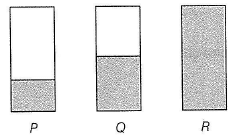Metals with the highest and lowest melting points respectively are

Detailed Solution for Test: Magnetic & Electric Properties of Solids & Semiconductors - Question 19

P indicates that bonding molecular orbitals are empty.

Q indicates that bonding molecular orbitals are filled.

R indicates that bonding and antibonding molecular orbitals are filled.

Highest melting point is for the metal having filled bonding molecular orbitals (they have maximum bond order). Lowest melting point is for the metal having filled bonding and anti-bonding molecular orbitals thus, bond order is zero. Increasing order of hardness of the metals is R < P< Q

Hardness increases with increasing bond order.

Test: Magnetic & Electric Properties of Solids & Semiconductors - Question 20

Passage

The following pictures show the electrons population of the composite s-d bands for three transition metals :Hardness of the metals in the increasing order is

Detailed Solution for Test: Magnetic & Electric Properties of Solids & Semiconductors - Question 20

P indicates that bonding molecular orbitals are empty.

Q indicates that bonding molecular orbitals are filled.

R indicates that bonding and antibonding molecular orbitals are filled.

Highest melting point is for the metal having filled bonding molecular orbitals (they have maximum bond order). Lowest melting point is for the metal having filled bonding and anti-bonding molecular orbitals thus, bond order is zero. Increasing order of hardness of the metals is

R < P< Q

Hardness increases with increasing bond order.

*Answer can only contain numeric values
Test: Magnetic & Electric Properties of Solids & Semiconductors - Question 21

Direction (Q. Nos. 21-24) This section contains 4 questions. When worked out will result in an integer from 0 to 9 (both inclusive).

Q.

How many of the following species show paramagnetism?

TiO, VO, V2O5 , NaCl, C6H6 , H2O, CH3COCH3, CuCl

Detailed Solution for Test: Magnetic & Electric Properties of Solids & Semiconductors - Question 21

(5)

Species with atieast one or more unpaired electron(s) are attracted by the magnetic field and is said to be paramagnetic.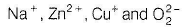No unpaired electron hence, diamagnetic.

O- Two unpaired electrons in anti bonding molecular orbitals.

Fe2+ - Four unpaired electrons in 3d-orbital.

Cr3+ - Three unpaired electrons in 3c/-orbital.

Cu+ - One unpaired electron in 3d-orbital.

O-2 One unpaired electron in antibonding molecular orbitals.

*Answer can only contain numeric values
Test: Magnetic & Electric Properties of Solids & Semiconductors - Question 22

Magnetic moment of a compound showing paramagnetism is 54.842 x 10-24 Am2 (Bohr magneton). How many unpaired electrons are there in the compound?

Detailed Solution for Test: Magnetic & Electric Properties of Solids & Semiconductors - Question 22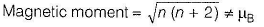where, n = number of unpaired electrons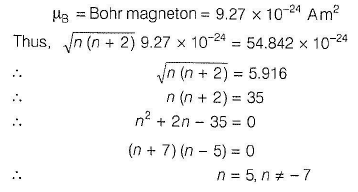Test: Magnetic & Electric Properties of Solids & Semiconductors - Question 23

How many of the following are diamagnetic in nature?

Detailed Solution for Test: Magnetic & Electric Properties of Solids & Semiconductors - Question 23

Diamagnetic substance have all paired electron.
(a) [Fe(CN)6​]3−Oxidation state of Fe=+3
It has one unpaired electron and is paramagnetic.
(b) NiCl42−​ - It has two unpaired electrons and is paramagnetic
(c) [Ni(CO)4​] - It has no unpaired electron and is diamagnetic
(d) [MnCl4​]2− - It is paramagnetic as it has five unpaired electrons.

*Answer can only contain numeric values
Test: Magnetic & Electric Properties of Solids & Semiconductors - Question 24

How many of the following are antiferromagnetic substances?

MnO, MnO2, Mn2O, FeO, Fe2O3, NiO ,Cr2O3, CoO, Co3O4 , H20, NaCl, C6H6

Detailed Solution for Test: Magnetic & Electric Properties of Solids & Semiconductors - Question 24

(9) Antiferromagnetism arises when net dipole alignment is zero due to equal and opposite alignment. This is observed in

MnO, MnO2, Mn2O, FeO, Fe2O3, NiO ,Cr2O3, CoO, Co3O4.

## Chemistry Class 12

150 videos|373 docs|213 tests
Information about Test: Magnetic & Electric Properties of Solids & Semiconductors Page
In this test you can find the Exam questions for Test: Magnetic & Electric Properties of Solids & Semiconductors solved & explained in the simplest way possible. Besides giving Questions and answers for Test: Magnetic & Electric Properties of Solids & Semiconductors, EduRev gives you an ample number of Online tests for practice

## Chemistry Class 12

150 videos|373 docs|213 tests

### How to Prepare for NEET

Read our guide to prepare for NEET which is created by Toppers & the best Teachers